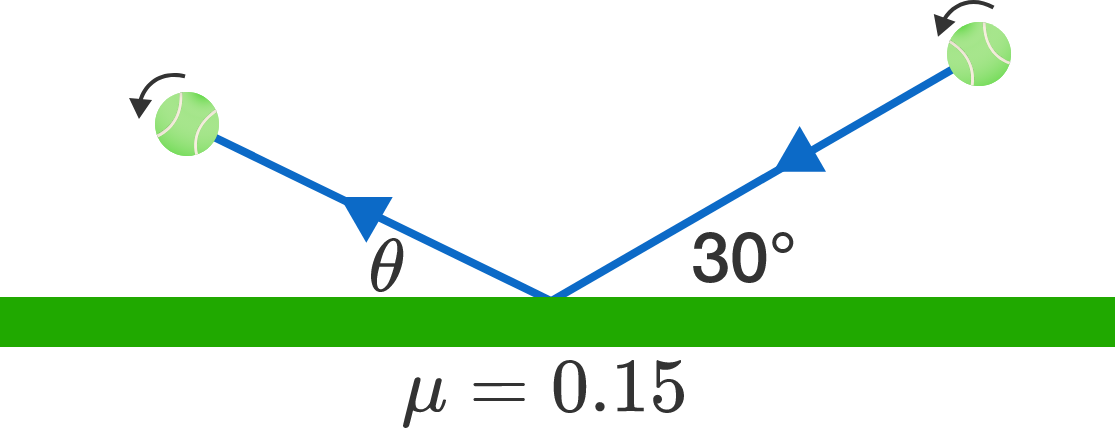# Top spin

A tennis ball hits the court with angle of incidence $30^\circ,$ and the ball has such a perfect top spin that even after it bounces, it is still spinning fast.

At what angle (in degrees relative to the horizontal) will the ball emerge after the collision?Details and Assumptions:

• A top spin means that the top of the ball moves in the same direction as the center of the ball, while the bottom of the ball moves in the opposite direction. For a really good top spin, the rotation of the ball is so fast that $\omega r \gg v$ is satisfied $(\omega$ is the angular velocity of the ball, $r$ is the ball's radius, and $v$ is the ball's velocity$).$
• Assume that the collision is as elastic as possible. The deformation of the ball during the collision does not take away any kinetic energy from the ball. On a perfectly slippery court, the ball would bounce back with the same kinetic energy.
• The coefficient of friction between the ball and the surface of the court is $0.15$.
×